### Home > PC > Chapter 4 > Lesson 4.2.4 > Problem4-115

4-115.
1. Simplify the following radical expressions. Homework Help ✎

1.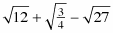2.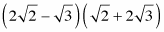3.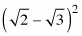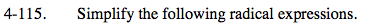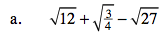Find perfect squares in each radical.

$\sqrt{(4)(3)}+\frac{\sqrt3}{2}-\sqrt{(9)(3)}$

Take the roots placing them outside of the radical.

$2\sqrt{3}+\frac{\sqrt3}{2}-3\sqrt3$

$\text{Combine the }\sqrt{3}\text{'s}$

$-\frac{\sqrt3}{2}$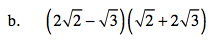Multiply the factors. Remember the middle terms.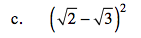$5-2\sqrt6$